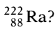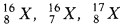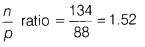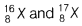# Find the atomic number

1. What is the n/p ratio of 2HAlso, find the atomic number.
2. Identify the pair of isotopes from the following:1. Number of neutrons, n =222 -88 = 134
Number of protons, p = 88Atomic number = number of protons = 88
2.are isotopes as they have same atomic number but different mass number.